# Magnetism

Certain metals and metallic oxides have the ability to attract other metals. This property is called magnetism, and the materials which have this property are called magnets. Some magnets are found naturally while others must be manufactured.

The following terms:

• Magnetic flux
• Magnetic flux density
• Weber
• Permeability
• Magnetomotive force (mmf)
• Ampere turns
• Field intensity
• Reluctance

#### Magnetism

Magnetism is a result of electrons spinning on their own axis around the nucleus (Figure 18).Figure 18 Electron Spinning Around Nucleus Produces Magnetic Field

In magnetic materials, the atoms have certain areas called domains. These domains are aligned such that their electrons tend to spin in the same direction (Figure 19).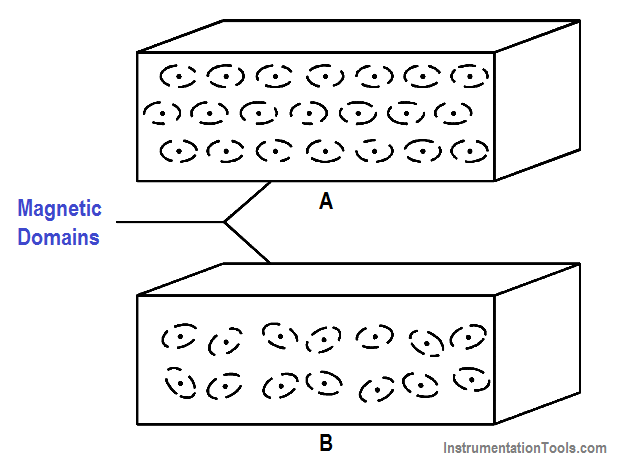Figure 19 Magnetic Domains

The alignment of these domains results in the formation of magnetic poles at each end of the magnet. These poles are called the north pole and the south pole. The law of magnetism states that like magnetic poles repel and unlike magnetic poles attract one another (Figure 20).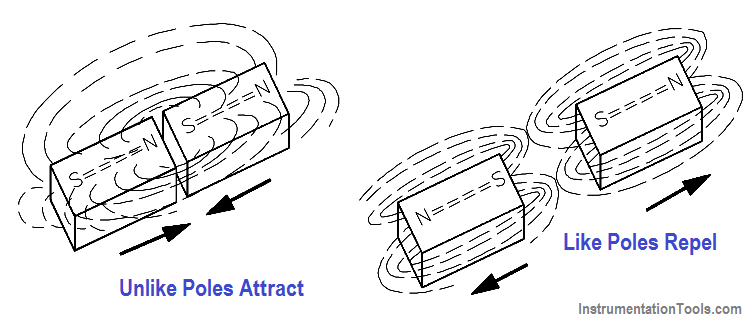Figure 20 The Law of Magnetic Attraction and Repulsion

#### Magnetic Flux

The group of magnetic field lines emitted outward from the north pole of a magnet is called magnetic flux. The symbol for magnetic flux is Φ (phi).

The SI unit of magnetic flux is the weber (Wb). One weber is equal to 1 x 108 magnetic field lines.

Example: If a magnetic flux (Φ) has 5,000 lines, find the number of webers.

Φ = 5000 lines / 1 x 108  lines/Wb = 5 x 10-6 Wb = 50 µ Wb

#### Magnetic Flux Density

Magnetic flux density is the amount of magnetic flux per unit area of a section, perpendicular to the direction of flux.

The below Equation is the mathematical representation of magnetic flux density.

B  = Φ / A

where

B = magnetic flux density in teslas (T)
Φ = magnetic flux in webers (Wb)
A = area in square meters (m2)

The result is that the SI unit for flux density is webers per square meter (Wb/m). One weber per square meter equals one tesla.

Example:  Find the flux density in teslas, when the flux is 800 µWb and the area is 0.004 m2

Given: Φ =800 µWb = 8×10-4 Wb

A = 0.0004 m2 =4 x 10-4 m2

B = Φ / A = (8×10-4 Wb) / (4 x 10-4 m2) = 2 Wb/m2

#### Magnetic Materials

Magnetic materials are those materials that can be either attracted or repelled by a magnet and can be magnetized themselves. The most commonly used magnetic materials are iron and steel. A permanent magnet is made of a very hard magnetic material, such as cobalt steel, that retains its magnetism for long periods of time when the magnetizing field is removed. A temporary magnet is a material that will not retain its magnetism when the field is removed.

Permeability (µ) refers to the ability of a material to concentrate magnetic lines of flux. Those materials that can be easily magnetized are considered to have a high permeability. Relative permeability is the ratio of the permeability of a material to the permeability of a vacuum (µo). The symbol for relative permeability is µR (mu).

Magnetic materials are classified as either magnetic or nonmagnetic based on the highly magnetic properties of iron. Because even weak magnetic materials may serve a useful purpose in some applications, classification includes the three groups described below.

Ferromagnetic Materials: Some of the ferromagnetic materials used are iron, steel, nickel, cobalt, and the commercial alloys, alnico and peralloy. Ferrites are nonmagnetic, but have the ferromagnetic properties of iron. Ferrites are made of ceramic material and have relative permeabilities that range from 50 to 200. They are commonly used in the coils for RF (radio frequency) transformers.

Paramagnetic Materials: These are materials such as aluminum, platinum, manganese, and chromium. These materials have a relative permeability of slightly more than one.

Diamagnetic Materials: These are materials such as bismuth, antimony, copper, zinc, mercury, gold, and silver. These materials have a relative permeability of less than one.

#### Electromagnetism

The relationship between magnetism and electrical current was discovered by a Danish scientist named Oersted in 1819. He found that if an electric current was caused to flow through a conductor, the conductor produced a magnetic field around that conductor (Figure 21).Figure 21 – The Magnetic Field Produced by Current in a Conductor

#### Polarity of a Single Conductor

A convenient way to determine the relationship between the current flow through a conductor and the direction of the magnetic lines of force around the conductor is the left-hand rule for current carrying conductors, as illustrated in Figure 22. The student should verify that the left hand rule holds true for the examples shown in Figure 21.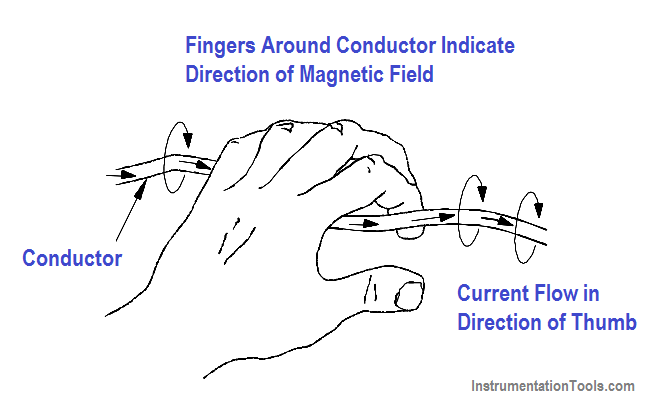Figure 22 – Left-hand Rule for Current Carrying Conductors

#### Magnetic Field and Polarity of a Coil

Bending a straight conductor into a loop has two results:

1. Magnetic field lines become more dense inside the loop, and
2. All lines inside the loop are aiding in the same direction.

When a conductor is shaped into several loops, it is considered to be a coil. To determine the polarity of a coil, use the left-hand rule for coils (Figure 23).Figure 23 – Left-hand Rule for Coils

Adding an iron core inside of a coil will increase the flux density. The polarity of the iron core will be the same as that of the coil. Current flow is from the negative side of the voltage source, through the coil, and back to the positive side of the source (Figure 24).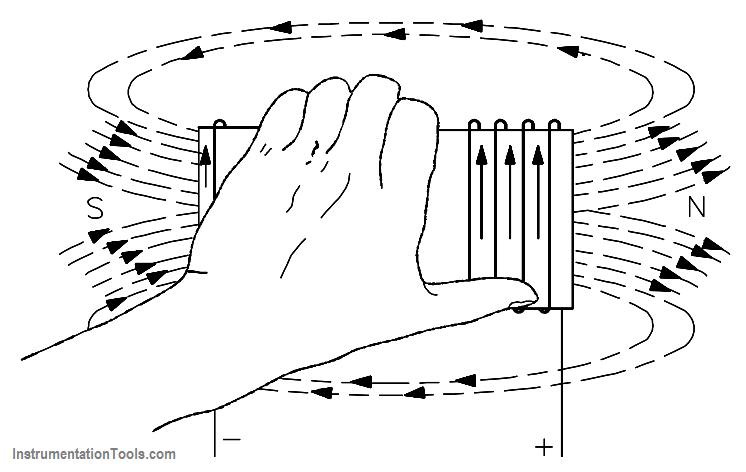Figure 24 – Left-hand Rule to Find North Pole of an Electromagnet

#### Magnetomotive Force

Magnetomotive force (mmf) is the strength of a magnetic field in a coil of wire. This is dependent on how much current flows in the turns of coil: the more current, the stronger the magnetic field; the more turns of wire, the more concentrated the lines of force. The current times the number of turns of the coil is expressed in units called “ampere-turns” (At), also known as mmf.

The below Equation is the mathematical representation for ampere-turns (At).

Fm = ampere-turns = NI

where

Fm = magnetomotive force (mmf)
N = number of turns
I = current

Example: Calculate the ampere-turns for a coil with 1000 turns and a 5mA current.

N = 1000 turns and I=5mA

substitute N = 1000 turns and I=5×10-3

NI = 1000 (5 x 10-3 ) = 5At

#### Field Intensity

When a coil with a certain number of ampere-turns is stretched to twice its length, the magnetic field intensity, or the concentration of its magnetic lines of force, will be half as great. Therefore, field intensity depends on the length of the coil.

The below Equation is the mathematical representation for field intensity, which is related to magnetomotive force as shown.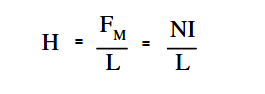where

H = field intensity, At/m
NI = ampere-turns (At)
L = length between poles of coil (m)
Fm = Magnetomotive force (mmf)

Example 1: Find field intensity of an 80 turn, 20 cm coil, with 6A of current.

Solution: N = 80, I = 6A, and NI = 480 At

H = 480 At / 0.2 m = 2400 At/m

Example 2: If the same coil in Example 1 were to be stretched to 40 cm with wire length and current remaining the same, find the new value of field intensity.

Solution: N = 80, I = 6A, and NI = 480 At

H = 480 At / 0.4 m = 1200 At/m

Example 3: The 20 cm coil used in Example 1 with the same current is now wound around an iron core 40 cm in length. Find the field intensity.

Solution: N = 80, I = 6A, and NI = 480 At

H = 480 At / 0.4 m = 1200 At/m

Note that field intensity for Examples 2 and 3 is the same.Figure 25 – Different Physical Forms of Electromagnets

#### Reluctance

Opposition to the production of flux in a material is called reluctance, which corresponds to resistance. The symbol for reluctance is R, and it has the units of ampere-turns per weber (At/wb).

Reluctance is related to magnetomotive force, mmf, and flux, Φ, by the relationship shown in equation.

R = mmf / Φ

Reluctance is inversely proportional to permeability (µ). Iron cores have high permeability and, therefore, low reluctance. Air has a low permeability and, therefore, a high reluctance.

Generally, different types of materials have different values of reluctance (Figure 25). Air gap is the air space between two poles of a magnet. Since air has a very high reluctance, the size of the air gap affects the value of reluctance: the shorter the air gap, the stronger the field in the gap. Air is nonmagnetic and will not concentrate magnetic lines. The larger air gap only provides space for the magnetic lines to spread out.

#### Summary

• Magnetic flux – group of magnetic field lines that are emitted outward from the north pole of a magnet
• Magnetic flux density – amount of magnetic flux per unit area of a section, perpendicular to the direction of the flux
• Weber – measure of magnetic flux
• Permeability – ability of a material to concentrate magnetic lines of flux
• Ferromagnetic materials – iron, steel, nickel, cobalt, and commercial alloys with relative permeability ranging from 50-200
• Paramagnetic materials – aluminum, platinum, manganese, and chromium with relative permeability of slightly more than one
• Diamagnetic materials – bismuth, antimony, copper, zinc, mercury, gold, and silver with relative permeability of less than one
• Magnetomotive force (mmf) – strength of a magnetic field in a coil of wire dependent on current flowing through coil
• Ampere turns – current flowing through a coil times the number of turns in the coil
• Field intensity – identifies the magnetic flux density per unit length of a coil
• Reluctance – opposition to the production of flux in a material